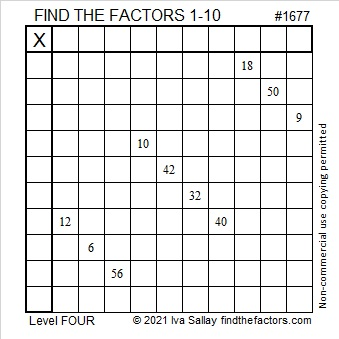# 1677 and Level 4

Contents

### Today’s Puzzle:

Using logic, write the numbers from 1 to 10 in both the first column and the top row so that this puzzle forms a multiplication table.### Factors of 1677:

• 1677 is a composite number.
• Prime factorization: 1677 = 3 × 13 × 43.
• 1677 has no exponents greater than 1 in its prime factorization, so √1677 cannot be simplified.
• The exponents in the prime factorization are 1, 1, and 1. Adding one to each exponent and multiplying we get (1 + 1)(1 + 1)(1 + 1) = 2 × 2 × 2 = 8. Therefore 1677 has exactly 8 factors.
• The factors of 1677 are outlined with their factor pair partners in the graphic below.### More About the Number 1677:

The last digit of every prime factor of 1677 is 3.

1677 is the hypotenuse of a Pythagorean triple:
645-1548-1677, which is (5-12-13) times 129.

This site uses Akismet to reduce spam. Learn how your comment data is processed.